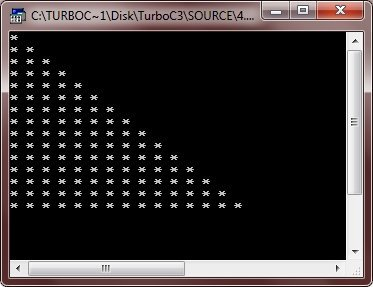# C++ Programming

## C++ Programming

### The While Loop

Introduction

The second loop available in C++ is the while loop. The while loop is an empty-controlled loop.

### C++ while Loop Syntax

Following is the syntax of the while loop :

```while(expression)
{
loop-body ;
}```

where the loop-body may contain a single statement, a compound statement or an empty statement. The loop iterates while the expression evaluates to true. When the expression becomes false, the program control passes to the line after the loop-body code.

In a while loop, a loop control variable should be initialized before the loop begins as an uninitialized variable can be used in the expression. The loop variable should be updated inside the body-of-the-while.

### C++ while Loop Example

Following example program illustrates the working of a while loop :

```/* C++ Iteration Statements - C++ while Loop */

#include<iostream.h>
#include<conio.h>
void main()
{
clrscr();
unsigned long num, fact=1;
cout<<"Enter a number: ";
cin>>num;
while(num)
{
fact = fact*num;
num--;
}
cout<<"The factorial of the number is "<<fact;
getch();
}```

When the above program compile and executed, it will produce the following output:The above program inputs an integer num. Then as long as num is nonzero (according to while (num)) the loop-body iterates i.e., fact is multiplied with num and the result is stored back in fact, followed by the decrement of num. Again the test-expression (num) is evaluated : if it is true, the loop repeated otherwise terminated.

## C++ do-while Loop

Unlike the for and while loops, the do-while is an exit-controlled loop i.e., it evaluates its test-expression at the bottom of the loop after executing its loop-body statements. This means that a do-while loop always executes at least once.

In the other two loops for and while, the test-expression is evaluated at the beginning of the loop i.e., before executing the loop-body. If the test-expression evaluates to false for the first time itself, the loop is never executed. But in some situations, it is wanted that the loop-body is executed at least once, no matter what the initial state of the test-expression is. In such cases, the do-while loop is the obvious choice.

### C++ do-while Loop Syntax

The syntax of the do-while loop is:

```do
{
statement ;
}while(test-expression);```

The braces { } are not necessary when the loop-body contains a single statement. The following do-while loop prints all upper-case letters :

```char ch = 'A' ;
do
{
cout << "\n" << ch ;
ch++;
}while(ch <= 'Z');```

The above code prints characters from ‘A’ onwards until the condition ch <= ‘Z’ becomes false.

### C++ do-while Loop Example

The most common use of the do-while loop is in menu selection routine, where the menu is flashed at least once. Then depending upon the user’s response it is either repeated or terminated.

The following example program is a menu selection program. Following program display a menu regarding rectangle operations and perform according to the user’s response :

```/* C++ Iteration Statements - C++ do-while Loop */

#include<iostream.h>
#include<conio.h>
#include<math.h>
#include<process.h>
void main()
{
clrscr();
char ch, ch1;
float l, b, peri, area, diag;
cout<<"\n 1. Area";
cout<<"\n 2. Perimeter";
cout<<"\n 3. Diagonal";
cout<<"\n 4. Exit\n";
do
{
cin>>ch;
if(ch == '1' || ch == '2' || ch == '3')
{
cin>>l>>b;
}
switch(ch)
{
case '1' : area = l * b ;
cout<<"Area = "<<area;
break ;
case '2' : peri = 2 * (l + b);
cout<<"Perimeter = "<<peri;
break;
case '3' : diag = sqrt((l * l) + (b * b));
cout<<"Diagonal = "<<diag;
break;
case '4' : cout<<"Breaking..Press a key..";
getch();
exit(1);
default : cout<<"Wrong choice !!!!";
cout<<"\nEnter a valid one";
break;
}     //end of switch
cout<<"\nWant to enter more (y/n) ? ";
cin>>ch1;
if(ch1 == 'y' || ch1 == 'Y')
cout<<"Again enter choice (1-4): ";
}while(ch1 == 'y' || ch1 == 'Y') ;      //end of DO-WHILE loop
getch();
}

```

## C++ Nested Loops

A loop may contain another loop in its body. This form of a loop is called nested loop. But in a nested loop, the inner loop must terminate before the outer loop. The following is an example of a nested loop.

```/* C++ Iteration Statements - C++ Nested Loops */

#include<iostream.h>
#include<conio.h>
void main()
{
clrscr();
int i, j;
for(i=0; i<15; i++)
{
for(j=0; j<=i; j++)
{
cout<<"* ";
}
cout<<"\n";
}
getch();
}```

When the above program is compile and executed it will produce the following output:Here is one more program, demonstrating nested loop in C++

```/* C++ Loops Program */

#include<iostream.h>
#include<conio.h>
void main()
{
clrscr();
int num, temp, i, j;
cout<<"Enter a number: ";
cin>>num;
for(i=0; i<10; i++)
{
num++;
temp=num;
for(j=0; j<=i; j++)
{
cout<<num<<" ";
num++;
}
cout<<"\n";
num=temp;
}
getch();
}```

Below is the sample run of the above C++ program:Now it’s time to do it yourself and feel free to mess around with the program to understand in-depth what each statement does. Don’t forget to save your program.

That’s it for today, see you next week!!!

` `